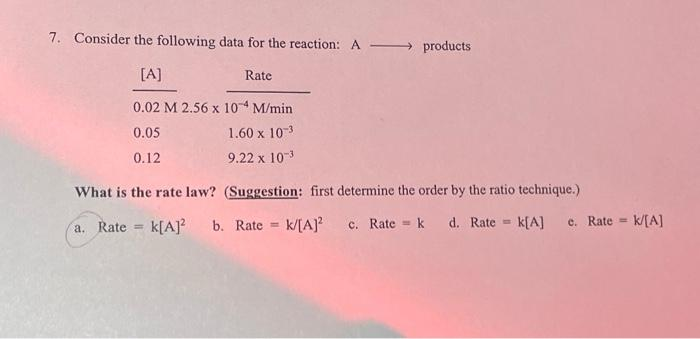Home / Expert Answers / Chemistry / 7-consider-the-following-data-for-the-reaction-a-products-a-0-02-m-2-56-x-10-4-m-min-0-05-pa380

# (Solved): 7. Consider the following data for the reaction: A products [A] 0.02 M 2.56 x 10-4 M/min 0.05 ...

7. Consider the following data for the reaction: A →→ products [A] 0.02 M 2.56 x 10-4 M/min 0.05 1.60 x 10-3 0.12 9.22 x 10-3 What is the rate law? (Suggestion: first determine the order by the ratio technique.) k[A]² b. Rate = k/[A]² c. Rate = k d. Rate = k[A] = a. Rate Rate = e. Rate = k/[A]7. Consider the following data for the reaction: products What is the rate law? (Suggestion: first determine the order by the ratio technique.) a. Rate b. Rate c. Rate d. Rate c.

We have an Answer from Expert